# Learning compositionality

This is a study project for the Introduction to Computational Neuroscience course at the University of Tartu

Team: Sander Sats, Tetiana Rabiichuk, Villem Tõnisson

Supervisors: Raul Vicente

January 28, 2021

# Initial description of our project

Operators such as AND, OR, NOT are fundamental to combine or compose different attributes in novel ways. Many newideas and concepts probably arise from combining other concepts or attributes using these operators (for example, you probablyhave never seen a penguin with glasses, but simply the words penguin AND glasses can make you imagine one). How the brainimplements such operations? In this project we will explore possible neuronal representations to enable such operations whichopen a huge combinatorial space.

The topic of the compositionality of previously learned concepts is a hot research topic in the machine learning community. One of the approaches to that problem uses energy-based models . The authors describe how they can combine independently learned concepts via logic operations on energy functions. They independently train energy functions for distinct concepts like curly hair, male, smiling, etc. Each function takes as input an image and produces an output depending how well the imagematches with the concept (c index i) of the function (if the concept is represented, the energy function value will be low, if not the valuewill be high). This is how they represent conditional distributions for an input image x bar:

For this to be a PDF (probability density function) it should integrate to 1, so there is also a normalization constant Z:

Though calculating this constant is often unfeasible as it requires integrating over the entire input domain. What this means is that if the value of the energy function is low, then the probability is high, and when the value of the energy function is high, the probability tends to zero. So in this case the image is a random variable, and we have a conditional distribution of concepts which is the probability that a given value of the random variable satisfies a concept (the likelihood that this image contains a specific concept, for example a smiling face) over the range of possible values of the random variable.

Having obtained these energy functions which represent distinct concepts independently (through learning or other means), if we want to find the probability of an instance to satisfy some concepts (c index 1 to k) simultaneously (concept conjunction: AND) we use the following formula:

# Primary theories

Humans can easily combine familiar concepts into novel ones without prior experience of such a concept combination. For instance: we can imagine a flying pig without ever seeing one (even if we know that pigs can’t fly). This task can be seen as the generation of a sample from some distribution. Also, whenever we imagine the same concept, we often get slightly different “mental images” (as samples from the distribution can and will vary due to the brain’s probabilistic nature).

We started our project by familiarizing ourselves with existing theories on how neurons can represent probability distributions, specifically with two of the main competing theories: sampling-based approach and probabilistic population coding (PPC). This was gruelling work as these concepts are heavy with mathematics and requirements for prior knowledge. One of the best sources for understanding probabilistic population code was reading chapters 6 and 7 in “Bayesian Brain: Probabilistic Approaches to Neural Coding” .

We also watched some conference talks on that topic (Mate Lengyel on sampling at Cosyne 2018  and CCN 2020 workshop on Probabilistic Computations in the Brain ) to wrap our heads around the topic. We were asked to work with gaussian distributions, which confused us about what should be the population (tuning curves, prior, and normalization factor) for the posterior to be a gaussian. The answer was found by reading “Probabilistic population codes and the exponential family of distributions” . This paper also helped us understand what is the “efficiency” of the PPC, and how PPC-s can be combined.

First let’s lay down some basic definitions that will be used going forward (not having a good place to look up definitions made reading all those papers much more difficult than it had to be):

• Firing rate — how many times a neuron spikes in a specific time span (for example 1 second).
• Mean firing rate — the average of a neuron’s instantaneous firing rates over multiple trials given the same stimulus.
• Tuning curve — the representations of a neuron’s mean firing rate depending on the stimulus (over the space of all possible stimuli).
• Uncertainty curve — how much the instantaneous firing rate can vary from trial to trial for the same neuron and same stimulus.
• Posterior — a function that describes the probabilities of all possible values of the stimulus given the observed response.
• Likelihood — a function that describes the probabilities of all possible neural activities given a stimulus value.
• Prior —a function that describes the probabilities of observing some stimulus before observing a given neural response. For the simplification of the calculations, prior knowledge is often assumed to be uniform, however, in the real world this is not the case. For instance, falling objects are far more likely to fall towards the ground. In lectures we have encountered some of the evidence towards the influence of the prior knowledge on our perception of the world, for instance, hollow face illusion.

Intuitively we see the difference between those two approaches as follows:

In the case of PPC, the population consists of many redundant neurons, each having their tuning curve high for some specific range of values of the stimulus and low for others. The value of the stimulus can be decoded by which neurons are firing most and the uncertainty of the result can be decoded by the differences of the firing rates of the spiking neurons in the population.

Whereas in the sampling case, the population is represented by one neuron. And the tuning curve determines a different firing rate for each value of the stimulus. So the value of the stimulus can be decoded from the firing rate and the uncertainty of the result can be decoded by the differences of firing rates from sampling the neuron multiple times with the same stimulus.

# Probabilistic Population Code (PPC)

In the classical approach to population coding the population encodes a stimulus value. The variability in neural response is considered a nuisance (noise) rather than a tool to represent the uncertainty. However, in recent years, a new approach has emerged: the variability in the response represents the environment’s stochastic nature and the uncertainty about the sensory information. For instance, the outside world is in a 3-dimensional space, while the retina can only represent the 2-dimensional projection of the input. There is an infinite number of possible 3-dim inputs that could generate such 2-dim projection.

We start off by describing population code in the traditional sense — as a way to encode stimulus value. Later we shift our focus to its probabilistic interpretation: the probabilistic population code and its applications.

## Population Code

It is easier to understand the idea behind population code on a concrete example. One of the classical ones is the encoding of the orientation s of a visual contour ranging from 0 to 180 degrees. Let’s assume our population consists of N neurons. Let’s consider an arbitrary neuron (n index i) from this population. We will consider the neuron’s activity at the firing rate level. For any particular stimulus value, this neuron has a mean firing rate that can be described by it’s tuning curve (f index i of s). One of the common assumptions is that a tuning curve is a bell-shaped function, for instance a Gaussian (see Figure 1). But we most probably never observe the mean response during an actual trial (presenting a stimulus). The firing rate of a neuron will vary and follow some distribution. If we were to fix the stimulus value and sample from the same neuron, we would get different firing rates, but the mean firing rate will be the value of that neuron’s tuning curve for that stimulus value. One of the simplest assumptions about the distribution of firing rates is that it follows a Poisson distribution since the Poisson distribution “expresses the probability of a given number of events occurring in a fixed interval of time or space if these events occur with a known constant mean rate and independently of the time since the last event”). Another simplification is that neural variability is independent. Poisson distribution is uniquely described by its lambda parameter, which is also equal to its mean and variance. Hence, for each fixed stimulus value s, the variability of a neuron follows a Poisson distribution (where lambda is the mean firing rate accoring to the neuron’s tuning curve for stimulus s). So the probability of observing a specific firing rate (r index i) given a stimulus value s is: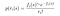Probability of observing a specifinc firing rate given a stimulus (4)

Then, the likelihood to observe a vector (r bar of length N) of response in a population of independent Poisson neurons is: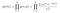Likelihood to observe a specific vector of response (5)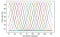Figure 1: Tuning curves of a homogeneous population of neurons with Gaussian tuning curves.

## Bayesian inference with Population Code

Next, we are going to explore the probabilistic nature of the population code. One can imagine the situation where it is useful to compute the posterior distribution over the possible stimulus value that elicited the response vector. For instance, when trying to catch a ball, we want to run towards the location it most likely will fall to. Hence, we want to know what is the current ball position and speed to calculate where it will fall. Thus, we would like to compute a distribution over possible stimulus values given the neural response. This information needs to be somehow represented in the brain to enable decision-making based on that information. By applying Bayes’ rule, we get:

Making the same assumptions about the population of neurons (Poisson variability, noise independence), and assuming a flat prior knowledge about stimulus distribution p(s) = 1 / L, one can show:

In the beginning, we also confused PPC with a Mixture of Experts, and we took the average over every neuron’s output. However, we were surprised to see that the average of the responses for various stimulus values is almost the same. Later we found the answer to the phenomena we have observed: that if the tuning curves of the neurons cover the stimulus range sufficiently densely, then their sum for any stimulus value is constant:

As each neuron is “an expert” in some range of stimulus value (around the peak of its tuning curve), then when we average, other neurons can only degrade the predictions of the “expert neurons” for that stimulus value. It was surprising to discover how the world of machine learning and neuroscience is interconnected. In machine learning, the PPC-like parametrization is known as a Product of Experts (was introduced by Geoffrey Hinton).

One of the questions that were bothering us while learning about PPC is: how do neurons “store” or “remember” mean firing rates, and how are those formulas (5, 7) actually “computed” by the neurons. It turns out that the PPC paradigm does not suggest that neurons implement Bayesian decoder explicitly; instead, the information stored by the neurons is enough to represent the posterior distribution. This view is different from the other theories of the uncertainty representation by neurons, where scientists try to directly match the neurons’ activity to the posterior distribution by linking the neural activity to either probability or log-probability of the stimulus value.

## Combining Population Codes

Let’s consider the following setting. A cat is chasing a rat. It wants to determine the rat’s location based on the sensory information: visual and auditory (this is called cue integration). Both sources encode the information about the same variable: the rat’s location. The unknown value of the stimulus in the probabilistic literature is referred to as a latent variable; in our example, the latent variable is the rat’s location. Three populations of neurons can perform the cue integration task. Let’s consider two populations of neurons:

• Population a encodes the rat’s location based on the visual information.
• Population b encodes the same rat’s location but relying on auditory cues.

Both populations encode independent information about the stimulus. From the simplification assumptions of independence of two populations and flat prior of possible rat’s location and using equations (6) and (7), we get:

The third population can combine both visual and auditory cues as follows:

For the following result, we need some simplification assumptions (some of them can be lifted). Let us assume that all three populations consist of the same number of Poisson neurons with independent noise. Moreover, population encoding cues have the same tuning curves. Given those assumptions, one can show a beautiful result: in order to combine the cues, all the third population has to do is to set its firing rate to be the sum of the other two population’s firing rates. And the third population (AND population) will have the same shape of tuning curves but with a larger amplitude. This result follows from equations (7) and (8):

From (9) it follows that if the tuning curves are Gaussian, then the posterior distribution is also Gaussian, since it will be quadratic in stimulus value:

And from (11) it follows that:

One can argue that neurons encode log-probabilities, since if we take the log of (10), we get a result proportional to r1 + r2, which is the information stored by the third population. When the assumption about Poisson variability of neurons is lifted, it can be shown that if the likelihood belongs to exponential family of functions with linear sufficient statistics, then PPC can express virtually any posterior distribution.

In the beginning each of us coded its own understanding of how the population coding works, so here are some exploratory coding examples (these are here for completeness sake, they are not finished works):

## Compositionality

The objective of our project was to figure what could be the possible neural implementations of concept conjunctions. For instance, following the cue integration example, if we have two populations of neurons, each encoding to one “concept,” for example, a “penguin” and “glasses,” how the third population can represent the AND of those two concepts: “penguin with glasses”. From what we can conclude from the literature we got familiar with, population coding is mostly used in perception-related settings. However, some researchers believe that PPC can be applied to learning as well. In case of the concept encoding it is now even clear how to represent its range of values.

After reading , we better understood what was the objective of our project. In the previous example, both populations encode the same random variable (the same location of the rat), however in case two populations encode two different “concepts”, then they encode random variables over two different domains. In order to simplify the notion of the concept, and to define the range of its values, we decided to work with concepts of “color” and “direction” of a line. Two populations will encode those concepts independently, one encoding color, and the other encoding direction. If we were to follow the framework of the population coding, then we need to figure out what tuning curves and what firing rates the third population has to choose to be able to restore the posterior distribution over color and direction. The authors of give a very nice answer in their assumptions (for the firing rates of the third population to be the sum of the firing rates of those two populations), but in their case the two populations encoded the same stimulus, so the posterior represented by the third population was 1-dimentional. But all the combining schemes we could think of, would increase the dimensionality. Unfortunately, we have not come up with the idea of how to solve this.

This is the colab where Sander tried to do some coding to wrap around the concepts but mostly failed (for completeness, messy and unfinished).

# Sampling

We did not look into sampling based approaches in as much depth as we did with the PPC, but in this section we will give a quick overview of how sampling-based approaches work and might be used in the brain.

Contrary to the PPC approach, neurons in the sampling approach represent the entire variable space of a variable for a concept. Instead of having multiple neurons that give output from which a probability distribution can be inferred, each neuron has its own probability distribution. Activity of these neurons corresponds to the value of the variable that they have a distribution about. This distribution is also changed by the incoming signal about the real world.

Firing rates corresponding to more probable world states would present themselves more often when sampled from a neuron. In the simplest case of this approach, we could say that the firing rate of a neuron is proportional to the value of the variable.

Given a sampling neuron with the probability distribution in figure 2, a possibility for the firing rates of 100 samples is seen in figure 3.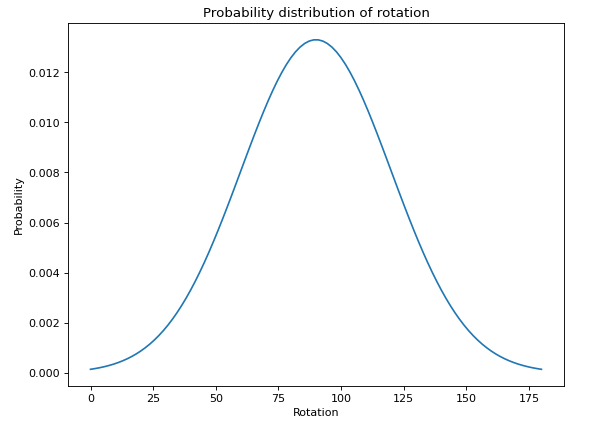Figure 2: Example of a probability distribution over rotation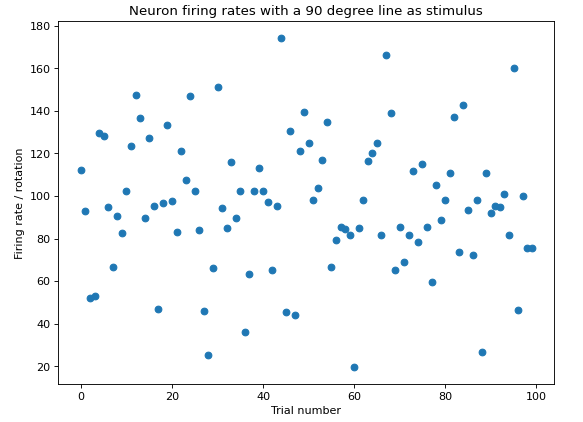Figure 3: Example of a neuron’s firing rates with a 90 degree line as stimulus

The main drawback of this method is the time that it takes to generate samples from the distribution. In PPC one sample from every neuron is enough to infer a probability distribution whereas in sampling, there would need to be more samples to get an accurate overview.

Sampling can also explain some spontaneous activity in the brain. Under a sampling-based representational account, spontaneous activity could have a natural interpretation. In a probabilistic framework, if neural activities represent samples from a distribution over external variables, this distribution must be the so-called ‘‘posterior distribution’’. The posterior distribution is inferred by combining information from two sources: the sensory input, and the prior distribution describing a priori beliefs about the sensory environment. Intuitively, in the absence of sensory stimulation, this distribution will collapse to the prior distribution, and spontaneous activity will represent this prior.

It is also possible to imagine how learning might be implemented in the case of sampling neurons. During off-line periods, such as sleeping, sampling from the prior could have a role in tuning synaptic weights thus contributing to the refinement of the internal model of the sensory environment.

In Figure 4 one can see a brief comparison of PPC and sampling-based approaches.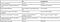Figure 4: Comparison of two main theories of representation of uncertainty with neurons

## Compositionality

We did not find much material about compositionality in the sampling-based approaches. There was an example in Figure 4 from  which shows how to calculate the output of two sampling neurons could be combined, but they offered no explanation of how this would be done on the neuronal level.

We came up with a method that could be used to infer the distribution of the AND function of two sampling neurons. This method only works if the probability distributions are about the same variable and are overlapping. The resulting distribution is also assumed to be a gaussian. It is quite simple to calculate and requires only a small of memory to be saved in neurons. This method functions similarly to some machine learning methods where the weights are changed iteratively for each incoming sample.

Lets say that we have a neuron X, that is in charge of creating a probability distribution from the outputs of 2 sampling neurons. This neuron X has internally saved 3 variables — the mean and the dispersion of a gaussian probability distribution. It has also saved a learning rate, which will be updated at the end of every timestep. At every timestep, this neuron receives inputs from 2 sampling neurons(the instantaneous firing rate) and updates the mean and dispersion according to the following formulae:

Where lr is the learning rate, mean is the mean saved in the neuron, dispersion is the dispersion of the distribution, alpha is a constant between 0 and 1 that shows the decay rate of the learning rate and sample1 and sample2 are firing rates from the sampling neurons.

One of the biggest weaknesses of using the sampling approach is the time that it takes to generate the samples. Using this method, the neuron would be able to create a probability distribution starting from the first samples. Another possible improvement is to start decreasing the variance of the gaussian, as more data comes in and the overlap of the two distributions become more clear. Once we need to use this neuron for creating another probability distribution, we would simply need to reset the learning rate and start feeding it new samples.

In practice, this method would probably require multiple neurons for the calculations and the saving of such information but it is a possibility to how neurons could create probability distributions from the outputs of sampling neurons. Instead of learning rate, the variables in the neuron could also change more based on the difference of current mean and the mean from the sample. The more the mean from the sample differs, the more we should move the mean saved in the neuron. This would enable the neuron to continuously work without requiring a resetting of the learning rate.

For example if we have 2 sampling neurons with separate probability distributions. One with mean of 20 and standard deviation of 10. The other with mean 40 and deviation of 5. We could impute AND of these two using the method described earlier. The result can be seen in Figure 5. In this figure, standard deviation of the inferred distribution was the cube root of the dispersion.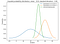Figure 5: How the output from two sampling neurons could be combined

The code for this approach is also available at the end of:

# Ideas how it could work but no idea how to implement

In this section are ideas that we were throwing around but couldn’t really implement or word correctly, so this should be taken with a couple of grains of salt.

They are loosely based on the idea of imagining. By imagining a concept, we can make the neurons that recognize that concept fire. Which means we can identify the populations and thus can reproduce the probability distributions and from those the likely world states that correspond to the imagined concept.

## Working backwards

Let’s say you want to imagine a red box, but you have never seen a red box. You have seen black box and a red ball though. Now you imagine the black box and mark all the neurons that were firing while you were imagining. Then you imagine the red ball and mark all the neurons that were firing while you were imagining. Now you take the neurons that got marked twice and work your way backwards from these neurons and calculate the input range where all these neurons fire. These are the world states corresponding to the concept of red box.

## Calculating intersection by using one PDF as the prior and the other as stimulus

Let’s say we are working with population codes that encode for parameters of a probability distribution. So given an input, the population will fire in a specific way to represent the probability distribution of world states that could cause the specific input.

Now let’s take two concepts c1 and c2 we know and get the relevant populations (population a and population b) that recognize these concepts (through imagination). Assuming we have a way to decode the probability distribution function from those populations, we decode the PDF-s f for a and g for b. Now we calculate the PDF h of their intersection by finding the high probability world states x of PDF f and calculating the high probability world states y of PDF g and then calculating the PDF of x in y. The world states that have a high probability of being in i are the same world states that have a high probability of satisfying concepts c1 and c2 (since they came from the high probability world states of populations that recognize those concepts) so h = i.

Note: We all read mostly the same academic papers and had many discussions where everyone contributed to the understanding of the topic.

Tetiana Rabiichuk: Contributed to the project report and presentation, Coding

Sander Sats: Ideas of how it could work, Formalization of blogpost, Coding

Villem Tõnisson: Sampling, Coding

# Aknowledgements

We would like to thank our supervisor Raul Vicente Zafra for his support and for introducing us to such an exciting topic.

The authors would like to thank European Regional Development Fund, the Archimedes Foundation, and the University of Tartu, for support by the Dora Scholarship and the University of Tartu for tuition waiver scholarship.

References:

 Ccn 2020 gac kickoff workshop 1 — probabilistic computations in the brain. https://www.youtube.com/watch?v=kn9f1fxxits.

 Hollow-face illusion. https://en.wikipedia.org/wiki/Hollow-Face_illusion.

 Poisson distribution. https://en.wikipedia.org/wiki/Poisson_distribution.

 J. Beck, W. Ma, P. Latham, and A. Pouget. Probabilistic population codes and the exponential family of distributions. In P. Cisek,T. Drew, and J. F. Kalaska, editors,Computational Neuroscience: Theoretical Insights into Brain Function, volume 165 ofProgress inBrain Research, pages 509–519. Elsevier, 2007.

 K. Doya, S. Ishii, A. Pouget, and R. Rao.Bayesian Brain: Probabilistic Approaches to Neural Coding. Computational neuroscience.MIT Press, 2007.

 Y. Du, S. Li, and I. Mordatch. Compositional visual generation and inference with energy based models.ArXiv, abs/2004.06030, 2020.

 J. Fiser, P. Berkes, G. Orb ́an, and M. Lengyel. Statistically optimal perception and learning: from behavior to neural representations.Trends in Cognitive Sciences, 14(3):119–130, Mar. 2010.

 G. E. Hinton. Products of experts. pages 1–6, 1999.

 G. E. Hinton. Training products of experts by minimizing contrastive divergence.Neural Comput., 14(8):1771–1800, Aug. 2002.

 M. Lengyel. Sampling: coding, dynamics, and computation in the cortex (cosyne 2018). https://www.youtube.com/watch?t=629&v=_WzVAbebFxw.

 A. Pouget, J. M. Beck, W. J. Ma, and P. E. Latham. Probabilistic brains: knowns and unknowns.Nature Neuroscience, 16(9):1170–1178,2013.